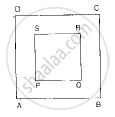# In the Given Figure, a Square Dart Board is Shown. the Length of a Side of the Larger Square is 1.5 Times the Length of a Side of the Smaller Square. If a Dart is Thrown and Lands on the L - Mathematics

Sum

In the given figure, a square dart board is shown. The length of a side of the larger square is 1.5 times the length of a side of the smaller square. If a dart is thrown and lands on the larger square. What is the probability that it will land in the interior of the smaller square?#### Solution

Given: A square dart board is shown. The length of a side of the larger square is 1.5 times the length of a side of the smaller square. If a dart is thrown and lands on the larger square

To find: Probability that it will land in the interior of the smaller square Let the length of smaller square is x cm Therefore the length of side of bigger square will be 1.5x cmArea of bigger square =(1.5x)^2

= 2.25x^2 cm^2

Area of smaller square=x^2cm^2

We know that Probability="Number of favourable event"/"Total number of event"

Hence probability that the dart will land in the interior of the smaller square is equal to =x^2/(2.25x^2)=4/9

Concept: Basic Ideas of Probability
Is there an error in this question or solution?